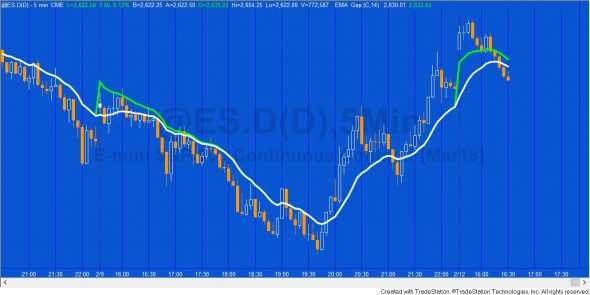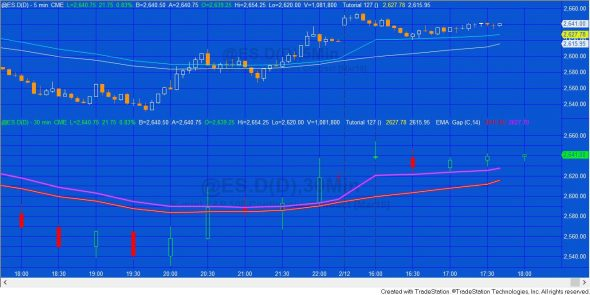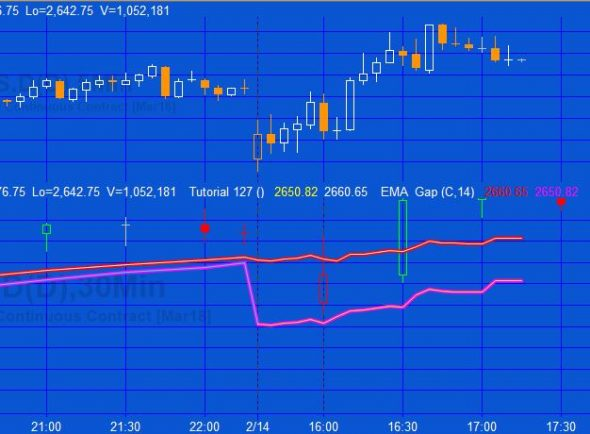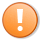# Tutorial 127 | Exponential Moving Averages with Price Gaps

## Improving the responsiveness of exponential moving averages when price gaps

Tutorial 127 explains techniques for modifying the exponential moving average (EMA) calculation to account for price gaps. The tutorial explains an approach for a simple EMA applied to a regular chart (tutorial program _EMA_Gap) and also the more complex situation when calculating the EMA of a price series provider (PSP) (N.B. PSP functionality is not available in MultiCharts.

The tutorial uses some of the same code as tutorial 86, however I have modified it significantly to take gaps into account. It would still be worth looking at tutorial 86 as background.A modified exponential moving average indicator applied to a 5 minute @ES.D chart demonstrating the difference between a standard EMA (in white) and one adjusted for gaps (in green). The tutorial program applied here is named _EMA_Gap (available free to Gold Pass members)

The image above shows a standard EMA in white and the one adjusted for gaps in green. Notice that the two EMAs converge during the day.The program described in this tutorial, running on the 5 minute chart, using a 30 minute PSP and applied to data 2 with an interval of 30 minutes. In this screen shot _EMA_Gap is applied to Data 2, using Data 2 data. _Tutorial127 is applied to Data 1 twice and displayed on both sub graph 1 and sub graph 2. If you click on the image to enlarge it and look closely at sub graph 2 you will see that the two indicators are overlayed exactly.

The above image shows a far more complex situation where both a ‘simple’ gap-adjusted EMA is calculated and plotted together with a far more complex PSP gap-adjusted calculation, i.e. rather than calculate the gap adjusted EMA based on the 30 minute bar data in data 2, the program calculates the equivalent EMA using a PSP when it is applied to 5 minute bars.

### What’s the point of using a Price Series Provider when you have data 2 loaded?

I utilized data 2 in the tutorial to demonstrate that the calculation from the simple EMA applied to data2 is the same as the calculation by the program using the smaller time frame in data1. _Tutorial127 does reference data2 BUT only to find the bar duration. This could easily be modified.On this screen shot the time before Feb 14 developed historically. The chart after developed in real time.

Although PSPs are very useful in allowing you to access data in situations where it would not normally be possible using multiple data streams, e.g. when using tick or volume charts and when using IntraBarOrderGeneration they also have some limitations such as the closing of PSP bars and Chart/RadarScreen bars are not synchronized, in real-time. In this tutorial you will sometimes see that the PSP bar close is different from the equivalent bar. This can cause some differences in the EMA between an EMA generated using the PSP and one developed using a data stream.

#### Programming lessons in _Tutorial127

The tutorial demonstrates how to:

• Utilize a price series provider
• Calculate an EMA with a PSP on a lower time frame chart
• Ensure that the EMA is updated, only when a PSP close update event occurs
• Modify the EMA calculation to adjust for a price gap after a new session begins.
• For a simple calculation
• For a PSP calculation
• To different calculations for real time and historic bars when using the PSP

### How _Tutorial127 works

Program instantiates a PSP using the time interval of data 2. It detects whether the symbols are set to local or exchange time and sets the PSP time to the same. It loads data going back to the datetime of the first bar of the chart and creates an update event.

The update event detects when the close of the PSP bar occurs.

On the first bar of the chart the normal EMA and the one that takes account of gaps are set to close.

The program detects when the session number changes and measures the value of the gap (if any).

#### For non-real time bars

For historic bars the program calculates a normal EMA and one that takes account of gaps. This is calculated on each bar but the equivalent of the EMA last bar is only set when a new PSP bar begins to form as determined by a change of the bar time. This is done by setting

`TimeLast = PSP.Time;`

within the subroutine and then checking when PSP.Time (the time of the PSP) is different.

#### For real-time bars

The real time formulae are the same only now we wait for a close update event of the PSP. At the next real time tick after this the equivalent of the EMA last bar is set.

The tutorial assumes that data1 is a minute bar with a duration that is shorter than the duration of data2 and which, in this tutorial, sets the PSP duration.

Tutorial 127 assumes that the EMA is calculated on the close.

After purchase you will be redirected to a download page, so don’t close the browser.

### Gold Pass areaThis content is for members only.

### Tutorial 127 | Video Part 2

A Gold Pass member asked how to remove the reference to data 2 from tutorial 127. In this second video I explain this.

Please let me know if you spot any bugs or errors or have questions.

THESE INDICATORS, SHOW ME STUDIES, STRATEGIES AND OTHER PROGRAMS HAVE BEEN INCLUDED SOLELY FOR EDUCATIONAL PURPOSES.

TO THE BEST OF MARKPLEX CORPORATION’S KNOWLEDGE, ALL OF THE INFORMATION ON THIS PAGE IS CORRECT, AND IT IS PROVIDED IN THE HOPE THAT IT WILL BE USEFUL. HOWEVER, MARKPLEX CORPORATION ASSUMES NO LIABILITY FOR ANY DAMAGES, DIRECT OR OTHERWISE, RESULTING FROM THE USE OF THIS INFORMATION AND/OR PROGRAM(S) DESCRIBED, AND NO WARRANTY IS MADE REGARDING ITS ACCURACY OR COMPLETENESS. USE OF THIS INFORMATION AND/OR PROGRAMS DESCRIBED IS AT YOUR OWN RISK.

ANY EASYLANGUAGE OR POWERLANGUAGE TRADING STRATEGIES, SIGNALS, STUDIES, INDICATORS, SHOWME STUDIES, PAINTBAR STUDIES, PROBABILITYMAP STUDIES, ACTIVITYBAR STUDIES, FUNCTIONS (AND PARTS THEREOF) AND ASSOCIATED TECHNIQUES REFERRED TO, INCLUDED IN OR ATTACHED TO THIS TUTORIAL OR PROGRAM DESCRIPTION ARE EXAMPLES ONLY, AND HAVE BEEN INCLUDED SOLELY FOR EDUCATIONAL PURPOSES. MARKPLEX CORPORATION. DOES NOT RECOMMEND THAT YOU USE ANY SUCH TRADING STRATEGIES, SIGNALS, STUDIES, INDICATORS, SHOWME STUDIES, PAINTBAR STUDIES, PROBABILITYMAP STUDIES, ACTIVITYBAR STUDIES, FUNCTIONS (OR ANY PARTS THEREOF) OR TECHNIQUES. THE USE OF ANY SUCH TRADING STRATEGIES, SIGNALS, STUDIES, INDICATORS, SHOWME STUDIES, PAINTBAR STUDIES, PROBABILITYMAP STUDIES, ACTIVITYBAR STUDIES, FUNCTIONS AND TECHNIQUES DOES NOT GUARANTEE THAT YOU WILL MAKE PROFITS, INCREASE PROFITS, OR MINIMIZE LOSSES.